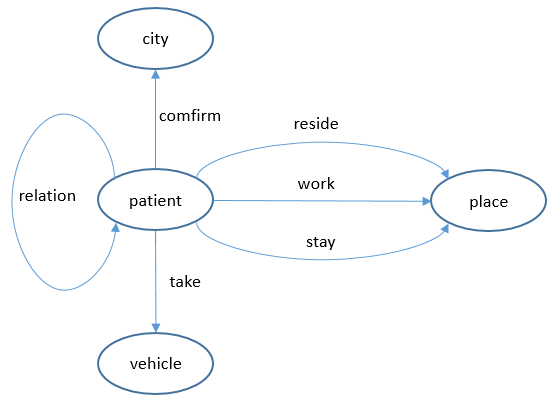# 從零開始學Graph Database：什麼是圖

## 基礎概念

### 什麼是圖？``````V:是節點的集合
E:是邊的集合
φ: E->{(x,y) | (x,y)∈ V^2 ∪ x≠y }是一個關聯函式，它每條邊對映到一個有頂點組成的有序對上。
````````````// 好友推薦邏輯

g.V("李雷").repeat(out("friend").simplePath().where(without('1hop')).store('1hop')).
times(2).path().by("name").limit(100)
``````

### 可以使用圖做什麼？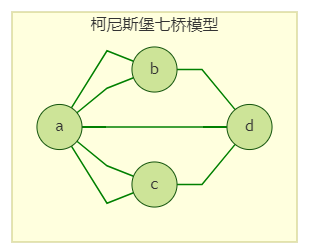``````連通的無向圖G有尤拉路徑（一筆畫）的充要條件是：G中的奇點的數目等於0或2。

（奇點：連線邊數為奇數的頂點。）
``````

#### 查詢與演算法

##### 圖查詢如：

1.gremlin

``````g.V("李雷").outE('friend').has('age',gt(30)).otherV().where(out('friend').
(hasId('李雷'))).limit(100)
``````

2.cypher

``````match (a)-[r1:friend]->(b)-[r2:friend]->(c) where a.mid='李雷' and r1.age>30 and a=c return id(b) limit 100
``````

##### 圖演算法實際的場景中，我們需要同時兼顧演算法的效果和執行成本。這也是很多使用場景所面臨的trade-off問題。正如我們前面所說，大部分情況下不需要用到非常高深的圖演算法，特別是在線上任務中，我們更看重時延和效率。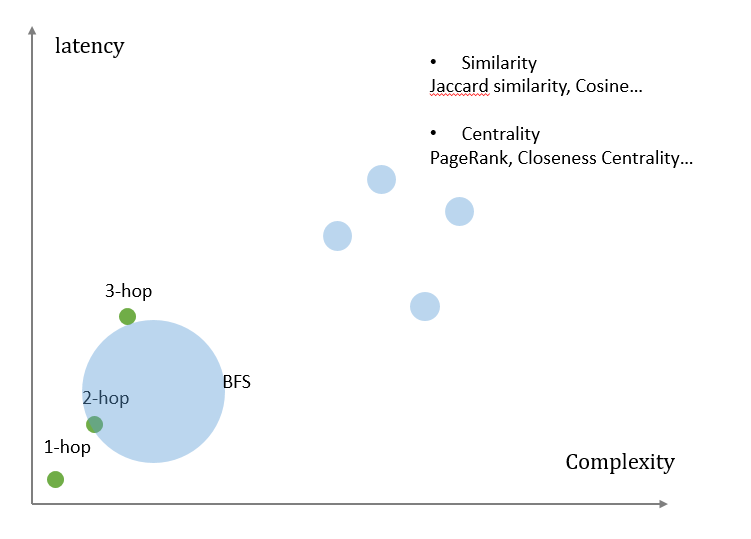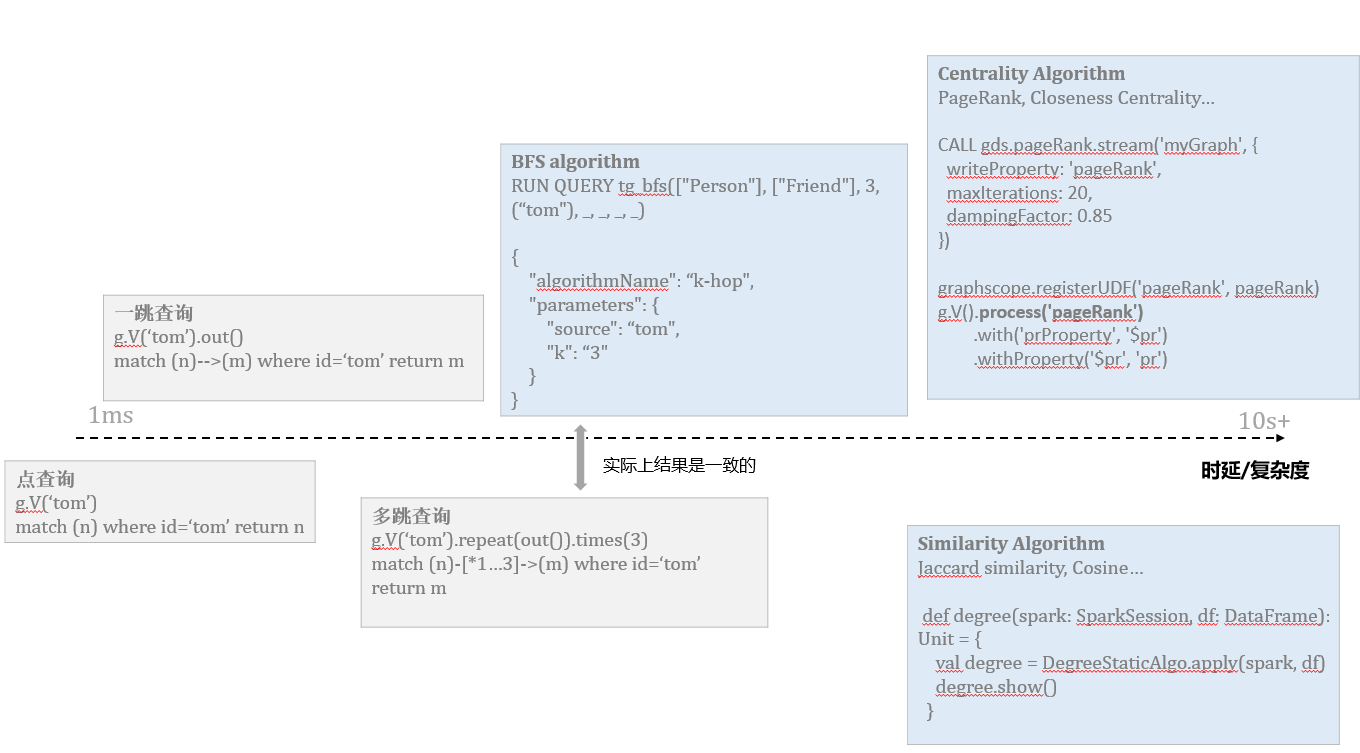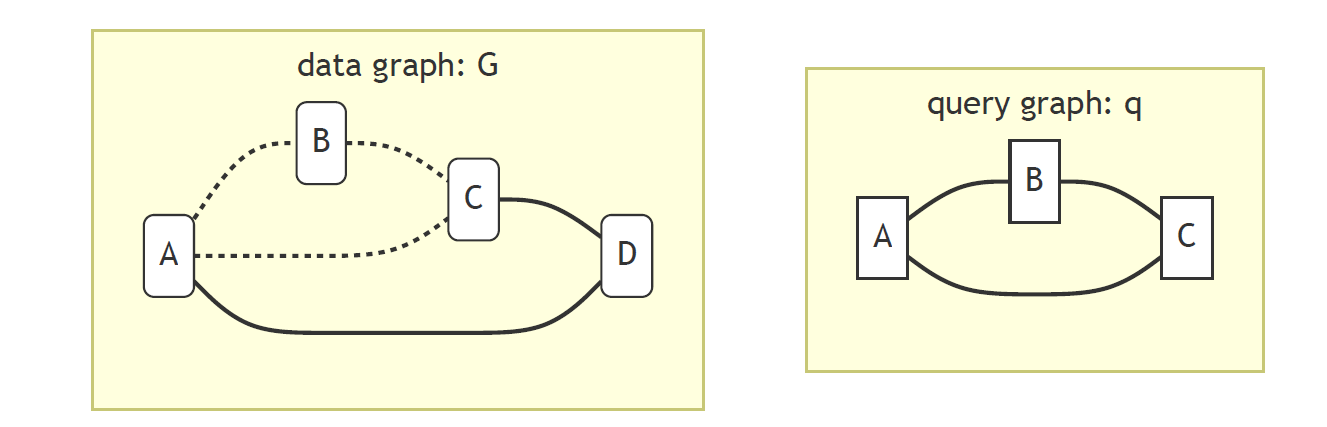圖的應用

``````gremlin: g.V('李雷').out().out()
cypher: match (n)--(m)--(l) where id(n)='李雷' return l
louvain:
[GES API]
POST /ges/v1.0/{project_id}/graphs/{graph_name}/action?action_id=execute-algorithm
{
"algorithmName": "louvain",
"parameters": {
"max_iterations": "100",
"convergence": "0.01",
"weight":"score"
}
}
``````